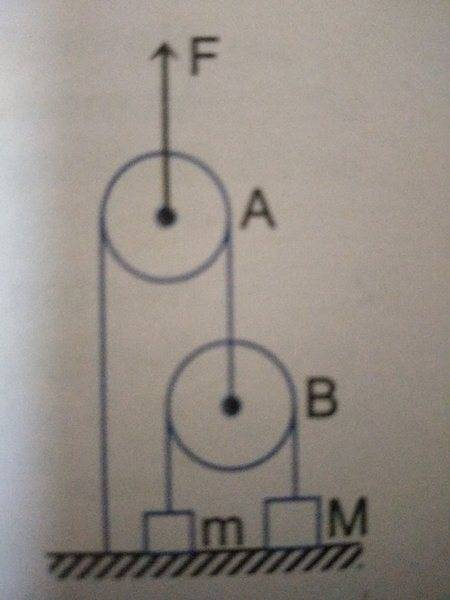# Constraint equations in mechanics

1. The problem: Two blocks of mass m=5kg and M= 10kg are connected by a string passing over a pulley B. Another string connects pulley B to the floor and passes over pulley A. An upward force F is applied at the centre of pulley A. Both pulleys are massless. Find acceleration of the blocks if F is:
a) 100N, b) 300N, c) 500N
Take g=10 ms-2

I have attached an image of the problem.

Attempt at a solution:
Well I drew an FBD in the frame of pulley A, thus using a downward pseudo force. Also, I found using constraint equations that F=4T, where T is the tension in the thread joining the two masses. Thus it gave me two equations ( one for each mass) but solving them, I found out that both the accelerations are turning out to be negative. Where did I go wrong?#### Attachments

Orodruin
Staff Emeritus
Homework Helper
Gold Member
2021 Award
Thus it gave me two equations ( one for each mass) but solving them, I found out that both the accelerations are turning out to be negative. Where did I go wrong?
How are we going to have any chance to answer that if you do not show us your calculations?

How are we going to have any chance to answer that if you do not show us your calculations?
I'm very sorry sir I'll put my attempt too

How are we going to have any chance to answer that if you do not show us your calculations?
I've now added my attempt too, sir

haruspex